Tag: Cuntz

Now
that my non-geometry
post
string-coffee-table post
which in turn is available through a
paper

The little
this : if you have a situation where a discrete group is acting with a
bad orbit-space (for example, $GL_2(\mathbb{Z})$ acting on the whole
complex-plane, rather than just the upper half plane) you can associate
to this a $C^*$-algebra and study invariants of it and interprete them
example is the one I mentioned and where the additional noncommutative
points (coming from the orbits on the real axis) seem to contain a lot
of modular information as clarified by work of Manin&Marcolli and
Zagier. Probably the best introduction into Connes-style
non-commutative geometry
from this perspective are the Lecture on
Arithmetic Noncommutative Geometry
by Matilde Marcolli. To
algebraists : this trick is very similar to looking at the
skew-group algebra $\mathbb{C}[x_1,\ldots,x_n] * G$ if
you want to study the _orbifold_ for a finite group action on affine
space. But as algebraist we have to stick to affine varieties and
polynomials so we can only deal with the case of a finite group,
analysts can be sloppier in their functions, so they can also do
something when the group is infinite.

By the way, the
skew-group algebra idea is also why non-commutative algebraic
geometry
enters string-theory via the link with orbifolds. The
easiest (and best understood) example is that of Kleinian singularities.
The best introduction to this idea is via the Representations
of quivers, preprojective algebras and deformations of quotient
singularities
notes by Bill Crawley-Boevey.

Artin-style non-commutative geometry aka
non-commutative projective geometry originated from the
work of Artin-Tate-Van den Bergh (in the west) and Odeskii-Feigin (in
the east) to understand Sklyanin algebras associated to elliptic curves
and automorphisms via ‘geometric’ objects such as point- (and
fat-point-) modules, line-modules and the like. An excellent survey
paper on low dimensional non-commutative projective geometry is Non-commutative curves and surfaces by Toby
Stafford and
Michel Van den Bergh
. The best introduction is the (also
neverending…) book-project Non-
commutative algebraic geometry
by Paul Smith who
maintains a
noncommutative geometry and algebra resource page
page (which is

Non-geometry
started with the seminal paper ‘Algebra extensions and
nonsingularity’, J. Amer. Math. Soc. 8 (1995), 251-289 by Joachim
Cuntz
and Daniel Quillen but which is not available online. An
online introduction is Noncommutative smooth
spaces
by Kontsevich and Rosenberg. Surely, different people have
different motivations to study non-geometry. I assume Cuntz got
interested because inductive limits of separable algebras are quasi-free
(aka formally smooth aka qurves). Kontsevich and Soibelman want to study
morphisms and deformations of $A_{\infty}$-categories as they explain in
their recent
paper
. My own motivation to be interested in non-geometry is the
hope that in the next decades one will discover new exciting connections
between finite groups, algebraic curves and arithmetic groups (monstrous
moonshine
being the first, and still not entirely understood,
instance of this). Part of the problem is that these three topics seem
to be quite different, yet by taking group-algebras of finite or
arithmetic groups and coordinate rings of affine smooth curves they all
turn out to be quasi-free algebras, so perhaps non-geometry is the
unifying theory behind these seemingly unrelated topics.

Here’s
an appeal to the few people working in Cuntz-Quillen-Kontsevich-whoever
noncommutative geometry (the one where smooth affine varieties
correspond to quasi-free or formally smooth algebras) : let’s rename our
topic and call it non-geometry. I didn’t come up with
this term, I heard in from Maxim Kontsevich in a talk he gave a couple
of years ago in Antwerp. There are some good reasons for this name
change.

The term _non-commutative geometry_ is already taken by
much more popular subjects such as _Connes-style noncommutative
differential geometry_ and _Artin-style noncommutative algebraic
geometry_. Renaming our topic we no longer have to include footnotes
(such as the one in the recent Kontsevich-Soibelman
paper
) :

We use “formal” non-commutative geometry
in tensor categories, which is different from the non-commutative
geometry in the sense of Alain Connes.

or to make a
distinction between _noncommutative geometry in the small_ (which is
Artin-style) and _noncommutative geometry in the large_ (which in
non-geometry) as in the Ginzburg notes.

Besides, the stress in _non-commutative geometry_ (both in Connes-
and Artin-style) in on _commutative_. Connes-style might also be called
‘K-theory of $C^*$-algebras’ and they use the topological
information of K-theoretic terms in the commutative case as guidance to
speak about geometrical terms in the nocommutative case. Similarly,
Artin-style might be called ‘graded homological algebra’ and they
use Serre’s homological interpretation of commutative geometry to define
similar concepts for noncommutative algebras. Hence, non-commutative
geometry is that sort of non-geometry which is almost
commutative…

But the main point of naming our subject
non-geometry is to remind us not to rely too heavily on our
(commutative) geometric intuition. For example, we would expect a
manifold to have a fixed dimension. One way to define the dimension is
as the trancendence degree of the functionfield. However, from the work
of Paul Cohn (I learned about it through Aidan Schofield) we know that
quasi-free algebras usually do’nt have a specific function ring of
fractions, rather they have infinitely many good candidates for it and
these candidates may look pretty unrelated. So, at best we can define a
_local dimension_ of a noncommutative manifold at a point, say given by
a simple representation. It follows from the Cunz-Quillen tubular
neighborhood result that the local ring in such a point is of the
form

$M_n(\mathbb{C} \langle \langle z_1,\ldots,z_m \rangle \rangle)$

(this s a noncommutative version of the classical fact
than the local ring in a point of a d-dimensional manifold is formal
power series $\mathbb{C} [[ z_1,\ldots,z_d ]]$) but in non-geometry both
m (the _local_ dimension) and n (the dimension of the simple
representation) vary from point to point. Still, one can attach to the
quasi-free algebra A a finite amount of data (in fact, a _finite_ quiver
and dimension vector) containing enough information to compute the (n,m)
couples for _all_ simple points (follows from the one quiver to rule them
all paper
or see this for more
details).

In fact, one can even extend this to points
corresponding to semi-simple representations in which case one has to
replace the matrix-ring above by a ring Morita equivalent to the
completion of the path algebra of a finite quiver, the _local quiver_ at
the point (which can also be computer from the one-quiver of A. The
local coalgebras of distributions at such points of
Kontsevich&Soibelman are just the dual coalgebras of these local
algebras (in math.RA/0606241 they
merely deal with the n=1 case but no doubt the general case will appear
in the second part of their paper).

The case of the semi-simple
point illustrates another major difference between commutative geometry
and non-geometry, whereas commutative simples only have self-extensions
(so the distribution coalgebra is just the direct sum of all the local
distributions) noncommutative simples usually have plenty of
non-isomorphic simples with which they have extensions, so to get at the
global distribution coalgebra of A one cannot simply add the locals but
have to embed them in more involved coalgebras.

The way to do it
is somewhat concealed in the
third version of my neverending book
(the version that most people
found incomprehensible). Here is the idea : construct a huge uncountable
quiver by taking as its vertices the isomorphism classes of all simple
A-representations and with as many arrows between the simple vertices S
and T as the dimension of the ext-group between these simples (and
again, these dimensions follow from the knowledge of the one-quiver of
A). Then, the global coalgebra of distributions of A is the limit over
all cotensor coalgebras corresponding to finite subquivers). Maybe I’ll
revamp this old material in connection with the Kontsevich&Soibelman
paper(s) for the mini-course I’m supposed to give in september.

or
rather, I’d like to subscribe to your brain! But I figure you’d allow
this (at best) only on a ‘share-alike’ basis so let me take the first
weblog, but what if you could be able to follow all traces I leave on
the web (or at least those you feel like following)? It’s a great idea
which started off with a couple of posts. Like John Resig’s Life as RSS

A little while ago I began to realize just how much of my personal
information is digitally created every day. This is both scary and
enticing (to me). Scary, due to the fact that people can harness this
information for evil/marketing. Enticing because I should be able to
(theoretically) harness this information to provide a better user
experience for the people who care (me and my friends, I assume). So,
the other day I sat down and tried to figure out every accessible data
… My masterplan:
Essentially, an RSS aggregator (makes sense, nothing special) that pulls
all of my personal RSS feeds into one place and provides an overall
statistical view of the information that it contains. I may even provide
some detailed information, save for things in the ‘Personal’ category.
completely public (or is possible to make completely public) – they’re
all using common/widely available programs or tools. So, stage one: Set
up a personal life browser – stage two: Open it up for the world to play
with.

soon to be followed upp by Lost Boy’s My Life in
RDF
and continued by Louche Cannon I want to subscribe

The other day I was talking to a former
colleague and I was trying to explain how I have gradually switched to
using an assortment of social content tools as my primary mechanism for
finding relevant and authoritative information on the web. With these
tools, I can subscribe to an assortment of RSS feeds produced by people
who I trust and think of as authorities in their respective subjects. In
short, I said, “I can subscribe to their brains”.
Or at least I
can in theory! At the moment, for those of non-geekly tendencies, the
practicalities of “subscribing to somebody’s brain” are a little
daunting. If you have an RSS-aware browser or have installed one of the
useful bookmarklets provided by the likes of bloglines, then subscribing
to individual RSS feeds is relatively easy. The problem is that I might
be interested subscribing to:
– What person X is blogging
– What person X is bookmarking- on several social bookmarking sites
(e.g. del.isio.us, CiteULike, Furl)
– What person X is listening
to (e.g. AudioScrobbler)
– What person X is taking pictures of
(e.g. Flickr)
– What person X’s travel schedule is (e.g. iCal)

– What books X is reading or planning on reading (e.g. Amazon
wish lists)
The first problem is finding out what feeds person
X provides. Most of the time you have to ask them, or search through the
individual services for the person’s name. If you are dealing with a
relatively clued-in person, you might be lucky enough to find links to
their various feeds off of their home page or in the margins of their
blogs. If you are dealing with an uber-geek, then you might find this
information encoded in their FOAF file. All that seems to be missing is
the button titled “Subscribe to X’s Brain”.

While it is
still a Work In Progress (and will continue to be for some time as I’d
like to get used to the idea and explore its possibilities) you’ll find
a button to ‘subscribe to my brain’ on the buttom left. Look out for
this :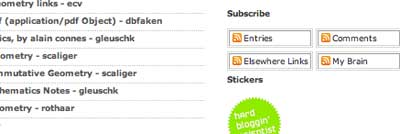Click on it and you’ll stare at a text-file. Save it to your desktop,
fire up your Newsaggregator (which I assume is something like NetNewsWire ). Look under
‘File’ for ‘Import Subscriptions’ and open the saved
BrainLeBruynL.opml-file. It will make a folder with name the present
date&time but you can always rename the folder to something like
‘Lieven’s brain’… Then you will look at something like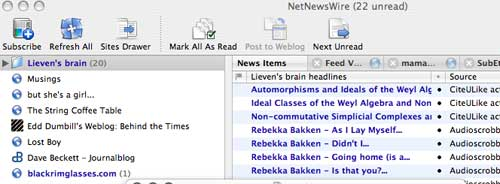which
will give you a pretty good idea of what I was upto just now (posting a
few references on Cuntz and Berest to CiteULike while listening to Rebekka Bakken via iTunes. If
you’ll open up the folder you get an even clearer picture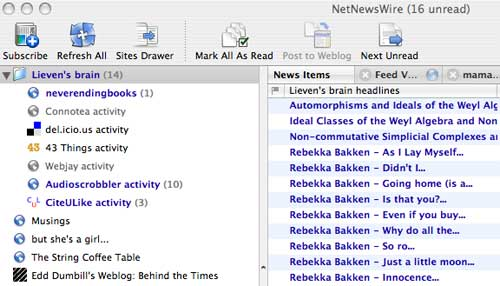which tells
you that since last time I’ve posted three new references to CiteULike,I
listened to at least 10 new songs (Audioscrobbler only remember the last
10 ones) and that there is one new post here! You can also check on my
recent bookmarks at del.icio.us and over the next few weeks you may also
detect activity in a few other places (and I may add an arXiv scraper
just in case you think I’m not posting there anymore). Clearly, it is
up to you to unsubscribe to those regions of my brain you don’t care to
follow but the overall picture may give you a pretty accurate picture of
my present ‘state of mind’. In the coming posts I’ll take you through
the process of setting up a ‘subscribe to my brain’ for yourself and
I’ll explore (for myself) some of the possible uses of this scheme. The
ultmate aim being to see buttons like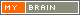appear on

A couple of days ago Ars Mathematica had a post Cuntz on noncommutative topology pointing to a (new, for me) paper by Joachim Cuntz

A couple of years ago, the Notices of the AMS featured an article on noncommutative geometry a la Connes: Quantum Spaces and Their Noncommutative Topology by Joachim Cuntz. The hallmark of this approach is the heavy reliance on K theory. The first few pages of the article are fairly elementary (and full of intriguing pictures), before the K theory takes over.

A few comments are in order. To begin, the paper is **not** really about noncommutative geometry a la Connes, but rather about noncommutative geometry a la Cuntz&Quillen (based on quasi-free algebras) or, equivalently, a la Kontsevich (formally smooth algebras) or if I may be so bold a la moi (qurves).

About the **intruiging pictures** : it seems to be a recent trend in noncommutative geometry research papers to include meaningless pictures to lure the attention of the reader. But, unlike aberrations such as the recent pastiche by Alain Connes and Mathilde Marcolli A Walk in the Noncommutative Garden, Cuntz is honest about their true meaning

I am indebted to my sons, Nicolas and Michael,
for the illustrations to the examples above. Since
these pictures have no technical meaning, they
are only meant to provide a kind of suggestive
visualization of the corresponding quantum spaces.

As one of these pictures made it to the cover of the **Notices** an explanation was included by the cover-editor

The image on this month’s cover arose from
Joachim Cuntz’s effort to render into visible art
his own internal vision of a noncommutative
torus, an object otherwise quite abstract. His
original idea was then implemented by his son
Michael in a program written in Pascal. More
explicitly, he says that the construction started
out with a triangle in a square, then translated
the triangle by integers times a unit along a line
with irrational slope; plotted the images thus
obtained in a periodic manner; and stopped
just before the figure started to seem cluttered.
Many mathematicians carry around inside
their heads mental images of the abstractions
they work with, and manipulate these objects
somehow in conformity with their mental imagery. They probably also make aesthetic judgements of the value of their work according to
the visual qualities of the images. These presumably common phenomena remain a rarely
explored domain in either art or psychology.

—Bill Casselman(covers@ams.org)

There can be no technical meaning to the pictures as in the Connes and Cuntz&Quillen approach there is only a noncommutative algebra and _not_ an underlying geometric space, so there is no topology, let alone a noncommutative topology. Of course, I do understand why Cuntz&others name it as such. They view the noncommutative algebra as the ring of functions on some virtual noncommutative space and they compute topological invariants (such as K-groups) of the algebras and interprete them as information about the noncommutative topology of these virtual and unspecified spaces.

Still, it is perfectly possible to associate to a qurve (aka quasi-free algebra or formally smooth algebra) a genuine noncommutative topological space. In this series of posts I’ll explain the little I know of the history of this topic, the thing I posted about it a couple of years ago, why I abandoned the project and the changes I made to it since and the applications I have in mind, both to new problems (such as the birational_classification of qurves) as well as classical problems (such as rationality problems for $PGL_n$ quotient spaces).

Although others have tried to define noncommutative topologies before, I learned about them from Fred Van Oystaeyen. Fred spend the better part of his career constructing structure sheaves associated to noncommutative algebras, mainly to prime Noetherian algebras (the algebras of preference for the majority of non-commutative algebraists). So, suppose you have an ordinary (meaning, the usual commutative definition) topological space X associated to this algebra R, he wants to define an algebra of sections on every open subset $X(\sigma)$ by taking a suitable localization of the algebra $Q_{\sigma}(R)$. This localization is taken with respect to a suitable filter of left ideals $\mathcal{L}(\sigma)$ of R and is defined to be the subalgebra of the classiocal quotient ring $Q(R)$ (which exists because $R$ is prime Noetherian in which case it is a simple Artinian algebra)

$Q_{\sigma}(R) = { q \in Q(R)~|~\exists L \in \mathcal{L}(\sigma)~:~L q \subset R }$

(so these localizations are generalizations of the usual Ore-type rings of fractions). But now we come to an essential point : if we want to glue this rings of sections together on an intersection $X(\sigma) \cap X(\tau)$ we want to do this by ‘localizing further’. However, there are two ways to do this, either considering $~Q_{\sigma}(Q_{\tau}(R))$ or considering $Q_{\tau}(Q_{\sigma}(R))$ and these two algebras are only the same if we impose fairly heavy restrictions on the filters (or on the algebra) such as being compatible.

As this gluing property is essential to get a sheaf of noncommutative algebras we seem to get stuck in the general (non compatible) case. Fred’s way out was to make a distinction between the intersection $X_{\sigma} \cap X_{\tau}$ (on which he put the former ring as its ring of sections) and the intersection $X_{\tau} \cap X_{\sigma}$ (on which he puts the latter one). So, the crucial new ingredient in a noncommutative topology is that the order of intersections of opens matter !!!

Of course, this is just the germ of an idea. He then went on to properly define what a noncommutative topology (and even more generally a noncommutative Grothendieck topology) should be by using this localization-example as guidance. I will not state the precise definition here (as I will have to change it slightly later on) but early version of it can be found in the Antwerp Ph.D. thesis by Luc Willaert (1995) and in Fred’s book Algebraic geometry for associative algebras.

Although _qurves_ are decidedly non-Noetherian (apart from trivial cases), one can use Fred’s idea to associate a noncommutative topological space to a qurve as I will explain next time. The quick and impatient may already sneak at my old note a non-commutative topology on rep A but please bear in mind that I changed my mind since on several issues…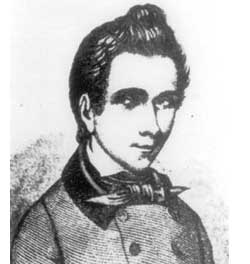Evariste Galois (1811-1832) must rank pretty high on the all-time
list of moving last words. Galois was mortally wounded in a duel he
fought with Perscheux d\’Herbinville on May 30th 1832, the reason for
the duel not being clear but certainly linked to a girl called
Stephanie, whose name appears several times as a marginal note in
Galois\’ manuscripts (see illustration). When he died in the arms of his
younger brother Alfred he reportedly said “Ne pleure pas, j\’ai besoin
de tout mon courage pour mourir ‚àö‚Ä† 20 ans”. In this series I\’ll
start with a pretty concrete problem in Galois theory and explain its
elegant solution by Aidan Schofield and Michel Van den Bergh.
Next, I\’ll rephrase the problem in non-commutative geometry lingo,
generalise it to absurd levels and finally I\’ll introduce a coalgebra
(yes, a co-algebra…) that explains it all. But, it will take some time
to get there. Start with your favourite basefield $k$ of
characteristic zero (take $k = \mathbb{Q}$ if you have no strong
preference of your own). Take three elements $a,b,c$ none of which
squares, then what conditions (if any) must be imposed on $a,b,c$ and $n \in \mathbb{N}$ to construct a central simple algebra $\Sigma$ of
dimension $n^2$ over the function field of an algebraic $k$-variety such
that the three quadratic fieldextensions $k\sqrt{a}, k\sqrt{b}$ and
$k\sqrt{c}$ embed into $\Sigma$? Aidan and Michel show in \’Division
algebra coproducts of index $n$\’ (Trans. Amer. Math. Soc. 341 (1994),
505-517) that the only condition needed is that $n$ is an even number.
In fact, they work a lot harder to prove that one can even take $\Sigma$
product
$A = k\sqrt{a} \ast k\sqrt{b} \ast k\sqrt{c}$ which is a pretty
monstrous algebra. Take three letters $x,y,z$ and consider all
non-commutative words in $x,y$ and $z$ without repetition (that is, no
two consecutive $x,y$ or $z$\’s). These words form a $k$-basis for $A$
and the multiplication is induced by concatenation of words subject to
the simplifying relations $x.x=a,y.y=b$ and $z.z=c$.

Next, they look
at the affine $k$-varieties $\mathbf{rep}(n) A$ of $n$-dimensional
$k$-representations of $A$ and their irreducible components. In the
parlance of $\mathbf{geometry@n}$, these irreducible components correspond
to the minimal primes of the level $n$-approximation algebra $\int(n) A$.
Aidan and Michel worry a bit about reducedness of these components but
nowadays we know that $A$ is an example of a non-commutative manifold (a
la Cuntz-Quillen or Kontsevich-Rosenberg) and hence all representation
varieties $\mathbf{rep}n A$ are smooth varieties (whence reduced) though
they may have several connected components. To determine the number of
irreducible (which in this case, is the same as connected) components
they use _Galois descent
, that is, they consider the algebra $A \otimes_k \overline{k}$ where $\overline{k}$ is the algebraic closure of
$k$. The algebra $A \otimes_k \overline{k}$ is the group-algebra of the
group free product $\mathbb{Z}/2\mathbb{Z} \ast \mathbb{Z}/2\mathbb{Z} \ast \mathbb{Z}/2\mathbb{Z}$. (to be continued…) A digression : I
cannot resist the temptation to mention the tetrahedral snake problem
in relation to such groups. If one would have started with $4$ quadratic
fieldextensions one would get the free product $G = \mathbb{Z}/2\mathbb{Z} \ast \mathbb{Z}/2\mathbb{Z} \ast \mathbb{Z}/2\mathbb{Z} \ast \mathbb{Z}/2\mathbb{Z}$. Take a supply of
tetrahedra and glue them together along common faces so that any
tertrahedron is glued to maximum two others. In this way one forms a
tetrahedral-snake and the problem asks whether it is possible to make
such a snake having the property that the orientation of the
\’tail-tetrahedron\’ in $\mathbb{R}^3$ is exactly the same as the
orientation of the \’head-tetrahedron\’. This is not possible and the
proof of it uses the fact that there are no non-trivial relations
between the four generators $x,y,z,u$ of $\mathbb{Z}/2\mathbb{Z} \ast \mathbb{Z}/2\mathbb{Z} \ast \mathbb{Z}/2\mathbb{Z} \ast \mathbb{Z}/2\mathbb{Z}$ which correspond to reflections wrt. a face of
the tetrahedron (in fact, there are no relations between these
reflections other than each has order two, so the subgroup generated by
these four reflections is the group $G$). More details can be found in
Stan Wagon\’s excellent book The Banach-tarski paradox, p.68-71.